# Common Core: High School - Geometry : Prove Parallelogram Theorems: CCSS.Math.Content.HSG-CO.C.11

## Example Questions

← Previous 1 3

### Example Question #1 : Prove Parallelogram Theorems: Ccss.Math.Content.Hsg Co.C.11

What is known ifis said to be a parallelogram?is a quadrilateral.

All of the other answers are correct.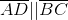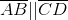Opposite side lengths are parallel.

All of the other answers are correct.

Explanation:

A parallelogram is a special type of quadrilateral meaning, it is a shape that has four sides with opposite sides being parallel.

Drawing the parallelogramcan be done as follows.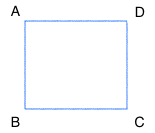Looking at the image it can be said that,and.

Therefore, all of the possible answer choices are correct.

### Example Question #103 : High School: Geometry

Which of the following helps prove an image is a parallelogram?

Two Lines must be Perpendicular

Congruent Opposite Angles

One Set of Parallel Lines

Angles that ExceedCongruent Opposite Angles

Explanation:

A parallelogram is a special type of quadrilateral meaning, it is a shape that has four sides with opposite sides being parallel. Along with opposite sides being congruent, a parallelogram has two pairs of opposite angles that are congruent. Lastly, the diagonals of a parallelogram must bisect each other.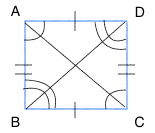### Example Question #1 : Prove Parallelogram Theorems: Ccss.Math.Content.Hsg Co.C.11

Which of the following helps prove an image is a parallelogram?

Angles that ExceedTwo Lines must be Perpendicular

One Set of Parallel Lines

Bisecting Diagonals

Bisecting Diagonals

Explanation:

A parallelogram is a special type of quadrilateral meaning, it is a shape that has four sides with opposite sides being parallel. Along with opposite sides being congruent, a parallelogram has two pairs of opposite angles that are congruent. Lastly, the diagonals of a parallelogram must bisect each other.### Example Question #2 : Prove Parallelogram Theorems: Ccss.Math.Content.Hsg Co.C.11

Which of the following helps prove an image is a parallelogram?

Two Sets of Opposite Parallel Lines

One Set of Parallel Lines

All of the answers are correct

Two Lines must be Perpendicular

Angles that ExceedTwo Sets of Opposite Parallel Lines

Explanation:

A parallelogram is a special type of quadrilateral meaning, it is a shape that has four sides with opposite sides being parallel. Along with opposite sides being congruent, a parallelogram has two pairs of opposite angles that are congruent. Lastly, the diagonals of a parallelogram must bisect each other.### Example Question #1 : Prove Parallelogram Theorems: Ccss.Math.Content.Hsg Co.C.11

Determine whether the statement is true or false:

A rhombus is an example of a parallelogram.

False

True

True

Explanation:

Recall that a parallelogram is a special type of quadrilateral meaning, it is a shape that has four sides with opposite sides being parallel. Along with opposite sides being congruent, a parallelogram has two pairs of opposite angles that are congruent. Lastly, the diagonals of a parallelogram must bisect each other. Since a rhombus has all of these characteristics it too is considered a parallelogram.

Therefore, the statement is true.

### Example Question #2 : Prove Parallelogram Theorems: Ccss.Math.Content.Hsg Co.C.11

Determine whether the statement is true or false:

A trapezoid is a parallelogram.

True

False

False

Explanation:

Recall that a parallelogram is a special type of quadrilateral meaning, it is a shape that has four sides with opposite sides being parallel. Along with opposite sides being congruent, a parallelogram has two pairs of opposite angles that are congruent. Lastly, the diagonals of a parallelogram must bisect each other.

A trapezoid has one set of opposite parallel sides however, they are not congruent. The opposite angles of a trapezoid are also not congruent therefore, the statement is false.

### Example Question #102 : Congruence

Determine whether the statement is true or false:

A quadrilateralhastherefore,is a parallelogram.

False

True

False

Explanation:

Recall that a parallelogram is a special type of quadrilateral meaning, it is a shape that has four sides with opposite sides being parallel. Along with opposite sides being congruent, a parallelogram has two pairs of opposite angles that are congruent. Lastly, the diagonals of a parallelogram must bisect each other.

Since the statement only says thatis an quadrilateral that has. This does not give enough information. A trapezoid has one pair of parallel sides but is not a parallelogram, therefore the statement, "A quadrilateralhastherefore,is a parallelogram." is false.

### Example Question #6 : Prove Parallelogram Theorems: Ccss.Math.Content.Hsg Co.C.11

Determine whether the statement is true or false:

In the figure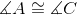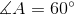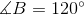, and opposite side lengths are congruent, thereforeis a parallelogram.

False

True

True

Explanation:

Recall that a parallelogram is a special type of quadrilateral meaning, it is a shape that has four sides with opposite sides being parallel and congruent. Along with opposite sides being congruent, a parallelogram has two pairs of opposite angles that are congruent. Lastly, the diagonals of a parallelogram must bisect each other.

Since the angles given represent those in a parallelogram, and the opposite sides are congruent, that makes the figure a parallelogram.

Therefore, the statement is true.

### Example Question #3 : Prove Parallelogram Theorems: Ccss.Math.Content.Hsg Co.C.11

Determine whether the statement is true or false.

Bisecting a parallelogram along one of its diagonals creates two congruent triangles.

True

False

True

Explanation:

Given the statement:

"Bisecting a parallelogram along one of its diagonal creates two congruent triangles."

Recall that a parallelogram is a special type of quadrilateral meaning, it is a shape that has four sides with opposite sides being parallel. Along with opposite sides being congruent, a parallelogram has two pairs of opposite angles that are congruent. Lastly, the diagonals of a parallelogram must bisect each other. When a parallelogram is bisected along one of its diagonals it in fact creates two congruent triangles.

Therefore, the statement is true.

### Example Question #101 : Congruence

Determine whether the statement is true or false:

To determine whether a figure is a parallelogram, you only need information on one pair of sides or one pair of angles.

True

False

False

Explanation:

Recall that a parallelogram is a special type of quadrilateral meaning, it is a shape that has four sides with opposite sides being parallel. Along with opposite sides being congruent, a parallelogram has two pairs of opposite angles that are congruent. Lastly, the diagonals of a parallelogram must bisect each other.

Since the statement gives one pair of sides and one pair of angles but does not specify whether the pairs are opposite it cannot be determined whether the figure is a parallelogram or not.

← Previous 1 3

### All Common Core: High School - Geometry Resources